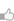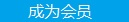求知 文章 文库 Lib 视频 iProcess 课程 角色 咨询 工具 讲座 Modeler Code要资料追随技术信仰 随时听讲座 每天看新闻

 Tensorflow教程 1. 新手入门 1. 1 介绍 1. 2 下载与安装 1. 3 基本用法 2. 完整教程 2. 1 总览 2. 2 MNIST 数据下载 2. 3 MNIST机器学习入门 2. 4 深入MNIST 2. 5 TensorFlow运作方式入门 2. 6 卷积神经网络 2. 7 字词的向量表示 2. 8 递归神经网络 2. 9 曼德布洛特集合 2. 10 偏微分方程 3 进阶指南 3. 1总览 3. 2变量:创建、初始化、保存和加载 3. 3TensorBoard:可视化学习 3. 4TensorBoard: 图表可视化 3. 5数据读取 3. 6线程和队列 3. 7增加一个新 Op 3. 8自定义数据读取 3. 9使用 GPUs 3. 10共享变量 4.资源 4.1总览 4.2BibTex 引用 4.3示例使用 4.4FAQ 4.5术语表 4.6 TENSOR排名、形状和类型

 932 次浏览49次捐助

 import tensorflow as tf import numpy as np # 使用 NumPy 生成假数据(phony data), 总共 100 个点. x_data = np.float32(np.random.rand(2, 100)) # 随机输入 y_data = np.dot([0.100, 0.200], x_data) + 0.300 # 构造一个线性模型 # b = tf.Variable(tf.zeros()) W = tf.Variable(tf.random_uniform([1, 2], -1.0, 1.0)) y = tf.matmul(W, x_data) + b # 最小化方差 loss = tf.reduce_mean(tf.square(y - y_data)) optimizer = tf.train.GradientDescentOptimizer(0.5) train = optimizer.minimize(loss) # 初始化变量 init = tf.initialize_all_variables() # 启动图 (graph) sess = tf.Session() sess.run(init) # 拟合平面 for step in xrange(0, 201): sess.run(train) if step % 20 == 0: print step, sess.run(W), sess.run(b) # 得到最佳拟合结果 W: [[0.100 0.200]], b: [0.300]您可以捐助，支持我们的公益事业。 1元 10元 50元 认证码：必填
 932 次浏览49次捐助每天2个文档/视频 扫描微信二维码订阅
 订阅技术月刊 获得每月300个技术资源